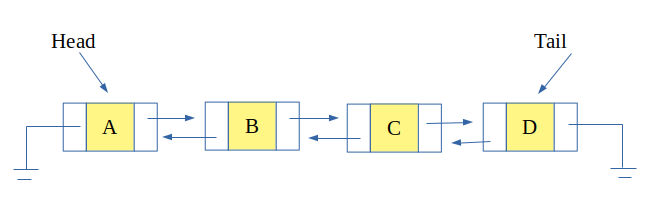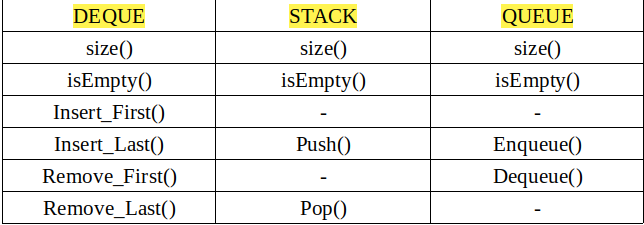# Implement Stack and Queue using Deque

Deque also known as double eneded queue, as name suggests is a special kind
of queue in which insertions and deletions can be done at the last as well
as at the beginning.

A link-list representation of deque is such that each node points to the next
node as well as the previous node. So that insertion and deletions take constant
time at both the beginning and the last.Now, deque can be used to implement a stack and queue. One simply needs to
understand how deque can made to work as a stack or a queue.

The functions of deque to tweak them to work as stack and queue are list below.Examples: Stack

```Input : Stack : 1 2 3
Push(4)
Output : Stack : 1 2 3 4

Input : Stack : 1 2 3
Pop()
Output : Stack : 1 2
```

Examples: Queue

```Input: Queue : 1 2 3
Enqueue(4)
Output: Queue : 1 2 3 4

Input: Queue : 1 2 3
Dequeue()
Output: Queue : 2 3
```

## Recommended: Please try your approach on {IDE} first, before moving on to the solution.

 `// CPP Program to implement stack and queue using Deque ` `#include ` `using` `namespace` `std; ` ` `  `// structure for a node of deque ` `struct` `DQueNode { ` `    ``int` `value; ` `    ``DQueNode* next; ` `    ``DQueNode* prev; ` `}; ` ` `  `// Implementation of deque class ` `class` `deque { ` `private``: ` ` `  `    ``// pointers to head and tail of deque ` `    ``DQueNode* head; ` `    ``DQueNode* tail; ` ` `  `public``: ` `    ``// constructor ` `    ``deque() ` `    ``{ ` `        ``head = tail = NULL; ` `    ``} ` ` `  `    ``// if list is empty ` `    ``bool` `isEmpty() ` `    ``{ ` `        ``if` `(head == NULL) ` `            ``return` `true``; ` `        ``return` `false``; ` `    ``} ` ` `  `    ``// count the number of nodes in list ` `    ``int` `size() ` `    ``{ ` `        ``// if list is not empty ` `        ``if` `(!isEmpty()) { ` `            ``DQueNode* temp = head; ` `            ``int` `len = 0; ` `            ``while` `(temp != NULL) { ` `                ``len++; ` `                ``temp = temp->next; ` `            ``} ` `            ``return` `len; ` `        ``} ` `        ``return` `0; ` `    ``} ` ` `  `    ``// insert at the first position ` `    ``void` `insert_first(``int` `element) ` `    ``{ ` `        ``// allocating node of DQueNode type ` `        ``DQueNode* temp = ``new` `DQueNode[``sizeof``(DQueNode)]; ` `        ``temp->value = element; ` ` `  `        ``// if the element is first element ` `        ``if` `(head == NULL) { ` `            ``head = tail = temp; ` `            ``temp->next = temp->prev = NULL; ` `        ``} ` `        ``else` `{ ` `            ``head->prev = temp; ` `            ``temp->next = head; ` `            ``temp->prev = NULL; ` `            ``head = temp; ` `        ``} ` `    ``} ` ` `  `    ``// insert at last position of deque ` `    ``void` `insert_last(``int` `element) ` `    ``{ ` `        ``// allocating node of DQueNode type ` `        ``DQueNode* temp = ``new` `DQueNode[``sizeof``(DQueNode)]; ` `        ``temp->value = element; ` ` `  `        ``// if element is the first element ` `        ``if` `(head == NULL) { ` `            ``head = tail = temp; ` `            ``temp->next = temp->prev = NULL; ` `        ``} ` `        ``else` `{ ` `            ``tail->next = temp; ` `            ``temp->next = NULL; ` `            ``temp->prev = tail; ` `            ``tail = temp; ` `        ``} ` `    ``} ` ` `  `    ``// remove element at the first position ` `    ``void` `remove_first() ` `    ``{ ` `        ``// if list is not empty ` `        ``if` `(!isEmpty()) { ` `            ``DQueNode* temp = head; ` `            ``head = head->next; ` `            ``head->prev = NULL; ` `            ``free``(temp); ` `            ``return``; ` `        ``} ` `        ``cout << ``"List is Empty"` `<< endl; ` `    ``} ` ` `  `    ``// remove element at the last position ` `    ``void` `remove_last() ` `    ``{ ` `        ``// if list is not empty ` `        ``if` `(!isEmpty()) { ` `            ``DQueNode* temp = tail; ` `            ``tail = tail->prev; ` `            ``tail->next = NULL; ` `            ``free``(temp); ` `            ``return``; ` `        ``} ` `        ``cout << ``"List is Empty"` `<< endl; ` `    ``} ` ` `  `    ``// displays the elements in deque ` `    ``void` `display() ` `    ``{ ` `        ``// if list is not empty ` `        ``if` `(!isEmpty()) { ` `            ``DQueNode* temp = head; ` `            ``while` `(temp != NULL) { ` `                ``cout << temp->value << ``" "``; ` `                ``temp = temp->next; ` `            ``} ` `            ``cout << endl; ` `            ``return``; ` `        ``} ` `        ``cout << ``"List is Empty"` `<< endl; ` `    ``} ` `}; ` ` `  `// Class to implement stack using Deque ` `class` `Stack : ``public` `deque { ` `public``: ` `    ``// push to push element at top of stack ` `    ``// using insert at last function of deque ` `    ``void` `push(``int` `element) ` `    ``{ ` `        ``insert_last(element); ` `    ``} ` ` `  `    ``// pop to remove element at top of stack ` `    ``// using remove at last function of deque ` `    ``void` `pop() ` `    ``{ ` `        ``remove_last(); ` `    ``} ` `}; ` ` `  `// class to implement queue using deque ` `class` `Queue : ``public` `deque { ` `public``: ` `    ``// enqueue to insert element at last ` `    ``// using insert at last function of deque ` `    ``void` `enqueue(``int` `element) ` `    ``{ ` `        ``insert_last(element); ` `    ``} ` ` `  `    ``// dequeue to remove element from first ` `    ``// using remove at first function of deque ` `    ``void` `dequeue() ` `    ``{ ` `        ``remove_first(); ` `    ``} ` `}; ` ` `  `// Driver Code ` `int` `main() ` `{ ` `    ``// object of Stack ` `    ``Stack stk; ` ` `  `    ``// push 7 and 8 at top of stack ` `    ``stk.push(7); ` `    ``stk.push(8); ` `    ``cout << ``"Stack: "``; ` `    ``stk.display(); ` ` `  `    ``// pop an element ` `    ``stk.pop(); ` `    ``cout << ``"Stack: "``; ` `    ``stk.display(); ` ` `  `    ``// object of Queue ` `    ``Queue que; ` ` `  `    ``// insert 12 and 13 in queue ` `    ``que.enqueue(12); ` `    ``que.enqueue(13); ` `    ``cout << ``"Queue: "``; ` `    ``que.display(); ` ` `  `    ``// delete an element from queue ` `    ``que.dequeue(); ` `    ``cout << ``"Queue: "``; ` `    ``que.display(); ` ` `  `    ``cout << ``"Size of Stack is "` `<< stk.size() << endl; ` `    ``cout << ``"Size of Queue is "` `<< que.size() << endl; ` `    ``return` `0; ` `} `

Output:

```Stack: 7 8
Stack: 7
Queue: 12 13
Queue: 13
Size of Stack is 1
Size of Queue is 1
```

Don’t stop now and take your learning to the next level. Learn all the important concepts of Data Structures and Algorithms with the help of the most trusted course: DSA Self Paced. Become industry ready at a student-friendly price.

My Personal Notes arrow_drop_upCheck out this Author's contributed articles.

If you like GeeksforGeeks and would like to contribute, you can also write an article using contribute.geeksforgeeks.org or mail your article to contribute@geeksforgeeks.org. See your article appearing on the GeeksforGeeks main page and help other Geeks.

Please Improve this article if you find anything incorrect by clicking on the "Improve Article" button below.

Article Tags :
Practice Tags :

1

Please write to us at contribute@geeksforgeeks.org to report any issue with the above content.